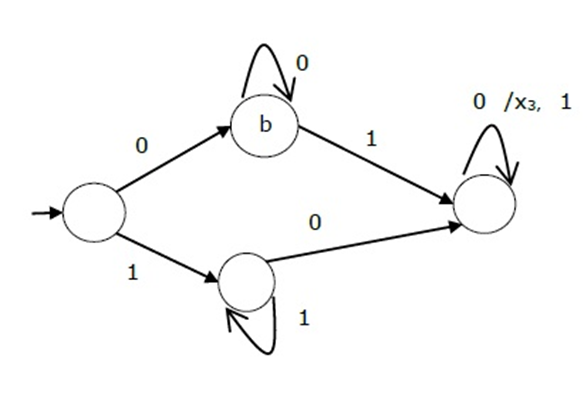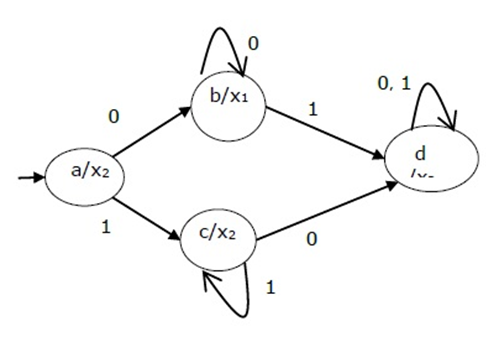# Comparative study of Mealy machine and Moore machine | TOC

TOC | Mealy machine Vs Moore machine: Here, we are going to learn what are Mealy machine and Moore machine? And what are the differences between Mealy machine and Moore machine in TOC?
Submitted by Mahak Jain, on November 12, 2018

Finite automata may also have outputs corresponding to each input symbol. Such finite automata are known as finite automata with the output.

There are two finite state machines with outputs namely Mealy machine and Moore machine.

### 1) Mealy machine

A finite state machine whose output generated depends on both the present state and the present input is called a mealy machine.

It is represented by 6 tuples (Q, ∑, O, δ, X, q0):

• Q is a set of states. It is finite in number.
• >∑ is a set of symbols called the input alphabets. They are also finite.
• O is a set of symbols called the output alphabets. They cannot be infinite in number.
• δ is the input transition function which maps Q × ∑ into Q.
• X is the output transition function which maps Q × ∑ into O.
• q0 is the initial start state from where any input starts getting processed (q0 ∈ Q).

### 2) Moore machine

Finite state machine whose output depends only on the present state.

It is represented by 6 tuples (Q, ∑, O, δ, X, q0):

• Q is a set of states. It is finite in number.
• is a set of symbols called the input alphabets. They are also finite.
• O is a set of symbols called the output alphabets. They cannot be infinite in number.
• δ is the input transition function where δ maps Q × ∑ into Q.
• X is the output transition function where X Maps Q into O.
• q0 is the initial start state from where any input starts getting processed (q0 ∈ Q).

## Comparison/Differences between Mealy machine and Moore machine

Mealy Machine Moore machine
Output depends on both upon present state and present input. Output depends only upon present state.
it has less states than Moore machine. it has more states than mealy machine.
It reacts faster to inputs. It reacts slower to inputs because more logic is required.
In same clock cycle. There are more circuit delays.
The output is placed on transitions. Output is placed on the states.
Less hardware is need to design. More hardware is required.
If there is a change in input then output also changes. There is no change in output if input changes.
It is very difficult to design. It is easy to design.What's New

Top Interview Coding Problems/Challenges!

Languages: » C » C++ » C++ STL » Java » Data Structure » C#.Net » Android » Kotlin » SQL
Web Technologies: » PHP » Python » JavaScript » CSS » Ajax » Node.js » Web programming/HTML
Solved programs: » C » C++ » DS » Java » C#
Aptitude que. & ans.: » C » C++ » Java » DBMS
Interview que. & ans.: » C » Embedded C » Java » SEO » HR
CS Subjects: » CS Basics » O.S. » Networks » DBMS » Embedded Systems » Cloud Computing
» Machine learning » CS Organizations » Linux » DOS
More: » Articles » Puzzles » News/Updates